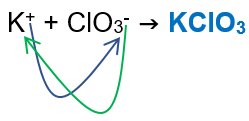# Problem: Determine the molality of a solution prepared by dissolving 10.66 g of potassium chlorate in 74.9 g of water. Give your answer to 2 decimal places.a. 0.14 mb .1.16 mc. 0.96 md .1.16x10-3 me. 1.40x103 m

###### FREE Expert Solution

We’re being asked to calculate for the molality of a solution prepared by dissolving 10.66 g of potassium chlorate in 74.9 g of water.

When calculating for molality, we use the following equation:

solution → water is the solvent (H2O)
solute → potassium chlorate

Potassium → Group 1A → +1 charge → K+
Chlorate → polyatomic ion → ClO3-

Potassium Chlorate:We will calculate the molality of the solution using the following steps:

82% (81 ratings)###### Problem Details

Determine the molality of a solution prepared by dissolving 10.66 g of potassium chlorate in 74.9 g of water. Give your answer to 2 decimal places.

a. 0.14 m
b .1.16 m
c. 0.96 m
d .1.16x10-3 m
e. 1.40x103 m